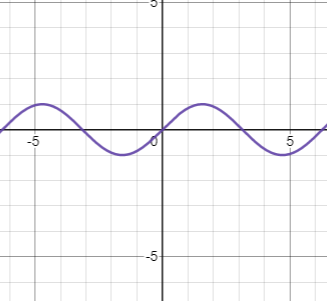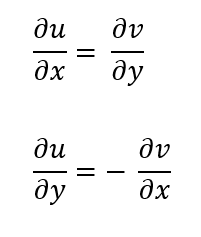Search
•Anand Zambare

# Complex numbers and complex analysis

"Humans create their own problems and then they find it difficult to deal with, for example, look at imaginary numbers (also known as complex numbers)." Surely lot of us have already seen this on any social media. It's funny right !. Taking away the fun part, have you ever given a thought to this? Why and how do complex numbers come into the existence? are they just a waste of time? Well thanks to Leonard Euler, his ideas of writing the i = sqrt(-1) proved us wrong. Let us explore some fundamentals of what complex numbers are and what is complex analysis.Consider the real number line which is shown in the figure below. We are well aware of this and we have used it. Complex numbers are plotted on a number line that is perpendicular to the real number line and that's why it is forming a complex plane. Im is the imaginary axis and Re is the usual real axis. The interesting point that you should consider is 'multiplication by i' to the existing number. So consider figure two, we can start by simply multiplying i with 1. we will get i in the first step, if we continue to multiply by i, we get -1, and in the next step we get -i. This continuation will make us trace a circle which is represented in the figure. So one can say that by multiplying with i we are basically rotating by 90 degrees.Figure 1 - Complex planeFigure 2 - Multiplying by i is rotation by 90 deg

So now we are well aware of what does multiplication by i mean physically. If we multiply by some factor of i say 2i or 3i or -4i it is scaling + rotation. Scaling is what we see in real numbers and rotation is because of the complex nature.

Once we understand the complex plane, it is easy to go ahead. Now any complex number is easy to locate on the plane. Say a+ib this is simply, a on the real axis and b on the imaginary axis. We won't be discussing any properties like magnitude or so as it is available in the textbook. This is more towards the physical sense of a complex field. Let's understand the complex functions now.

Complex functions and the analysis of complex functions have a lot of applications in the solutions of differential equations for example use of complex functions is done for transformations of the coordinates so we need to take out that perspective of saying 'Imaginary' meaning it doesn't exist actually. Consider a simple complex function (it does sound contradictory I know !) which is defined as the exponential of (ix). This is simplified by Euler's formula which saysEuler's formula

so if we go on and visualize the function in a complex plane we will see cosine on the real part and sine on the imaginary part together when extended forming the helical structure.Sine wave on imaginary axisCos wave on real axis

Once we can understand the complex plane, understanding the complex functions get easy. It is clearly evident that any complex function F(z) can be written as linear combination of function u & v i.e. written as F(z) = u + iv. u and v are separate functions of say x. Limits, derivatives, integrals all of the operations that are defined for the real functions are also defined for the complex functions.

(Note: All the three operations defined here are defined assuming that you are already familiar with the idea of these operations in real functions)

A) Limits :

The problem of finding the limits is very easy to deal with in the case of complex numbers if you have some background in limits for real numbers. As F(z) = u+iv while taking the limits we can separately take limits for u and v. Since i = sqrt(-1) which basically is constant there is absolutely no issue when it comes to dealing with limits.B) Differentiation :

The differentiation should be done using the same rule as we do for real functions. Differentiate u and v separately. The continuity of the derivatives is always what we look for. The Cauchy-Riemann equations play an important role in this. For F(z) to be a complex differentiable the function F(z) should satisfy Cauchy-Riemann i.e. C-R equations. The following equations are called C-R equations:Cauchy-Riemann equations

If and only if F(z) satisfies the C-R equations it is a complex differential. It is also known as Analytic functions.

C) Integration :

Only analytic functions in complex planes can be integrated. There are different ways of finding out the integrals and the residue method is one of the popular methods for solving the integrals of complex functions. We will look at the residue method in upcoming blogs.

The topics under the analysis of complex functions are quite interesting to understand practically. We will explore the topic of conformal mappings which has a lot of applications in the field of fluid mechanics and heat transfer as we move to the next blog. There is some awesome history behind the discovery of complex numbers, history is always fascinating!

History of Complex Numbers:

Back in the 14th-15th century, numbers were invented and some operations were known as subtraction, addition or so. Such invention was the need of the hour at that time. To quantify the daily stuff like distance, volume or so. But in the beginning, nobody believed in the real negative numbers. Geometry was an integral part of algebra itself. The logic they used to argue was simple and straight forward that there is no geometrical meaning to the negative length for example, what does it mean geometrically to have -2 meters long rod.

Soon people started to work with algebra separately and found that the quadratic equations are something that they will come across a lot of time. Now they had realized that the general solution in such a situation is required. So a lot of people started to work on finding the solution of a general quadratic equation which is a.(x^2)+b.x+c = 0. Soon the progression of this happened. now people were working on finding the general solution for cubic equations. (Just a little correction here by people I mean mathematicians only)

This was the point where mathematicians were stuck. They were clueless about what can be done. Few people worked out the way to solve the cases with only positive coefficients. The next big thing that came was the algorithm to solve for depressed cubic equations. Depressed cubic equations are cubic equations in which the square term of the unknown variable is not present. So the development of this algorithm is also known as Cardano's method.

Cardano's method is actually used to convert first the general equation into the depressed equation and then solve it as a depressed equation. But what happened is, people, realized over the period of time that most solutions include the square root of the negative numbers. Sooner the realization of imaginary numbers came into the play. It took 2 centuries from 14th to the 16th century for the realization of this. Leonard Euler was the big revolutionary name in the world of imaginary numbers. He not only proposed the complex functions but also developed the techniques to integrate and differentiate such functions. His work led the foundation for the transformation techniques which we will see soon in the upcoming blogs.

This Youtube channel "Veritasium" is really an awesome channel. If you are interested in knowing more about the history of the discovery of complex numbers, please do watch this linked video. https://youtu.be/cUzklzVXJwo